# Ball Mill Design/Power Calculation

The basic parameters used in ball mill design (power calculations), rod mill or any tumbling mill sizing are; material to be ground, characteristics, Bond Work Index, bulk density, specific density, desired mill tonnage capacity DTPH, operating % solids or pulp density, feed size as F80 and maximum ‘chunk size’, product size as P80 and maximum and finally the type of circuit open/closed you are designing for.

Use this online calculators for Motor Sizing and Mill sizing as well as mill capacity determinators.

In extracting from Nordberg Process Machinery Reference Manual I will also provide 2 Ball Mill Sizing (Design) example done ‘by-hand’ from tables and charts. Today, much of this mill designing is done by computers, power models and others.  These are a good back-to-basics exercises for those wanting to understand what is behind or inside the machines.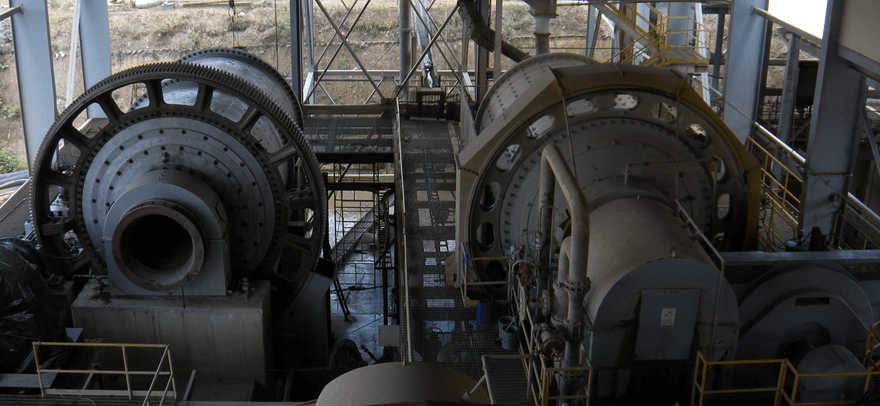The power required to grind a material from a given feed size to a given product size can be estimated by using the following equation: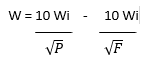where:

W = power consumption expressed in kWh/short to (HPhr/short ton = 1.34 kWh/short ton)
Wi = work index, which is a factor relative to the kwh/short ton required to reduce a given material from theoretically infinite size to 80% passing 100 microns
P = size in microns of the screen opening which 80% of the product will pass
F = size in microns of the screen opening which 80% of the feed will pass

When the above equation is used, the following points should be borne in mind:

• The values of P and F must be based on materials having a natural particle size distribution.
• The power consumption per short ton will only be correct for the specified size reduction when grinding wet in closed circuit. If the method of grinding is changed, power consumption also changes as follows:
1. Closed Circuit = W
2. Open Circuit, Product Top-size not limited = W
3. Open Circuit, Product Top-size limited = W to 1.25W

Open circuit grinding to a given surface area requires no more power than closed circuit grinding to the same surface area provided there is no objection to the natural top-size.  If top-size must be limited in open circuit, power requirements rise drastically as allowable top-size is reduced and particle size distribution tends toward the finer sizes.

• The work index, Wi, should be obtained from test results or plant data, where the feed and product size distributions are as close as possible to those under sturdy.
The work index, Wi, will vary considerably for materials that appear to be very similar. The work index will also have a considerable variation across one ore body or deposit.
The most reliable work index values are those obtained from long term operating data. If this is not available, standard grindability tests can be run to provide approximate values.
Rod and ball mill grindability test results should only be applied to their respective methods of grinding.

Ball Mill Power Calculation Example #1

A wet grinding ball mill in closed circuit is to be fed 100 TPH of a material with a work index of 15 and a size distribution of 80% passing ¼ inch (6350 microns). The required product size distribution is to be 80% passing 100 mesh (149 microns). In order to determine the power requirement, the steps are as follows:

A motor with around 1400 Horse Power is calculated needed for the designed task.  Now we much select a Ball Mill that will draw this power.

The ball mill motor power requirement calculated above as 1400 HP is the power that must be applied at the mill drive in order to grind the tonnage of feed from one size distribution. The following shows how the size or select the matching mill required to draw this power is calculated from known tables ‘the old fashion way’.

Here is a section of a mill in operation. The power input required to maintain this condition is theoretically: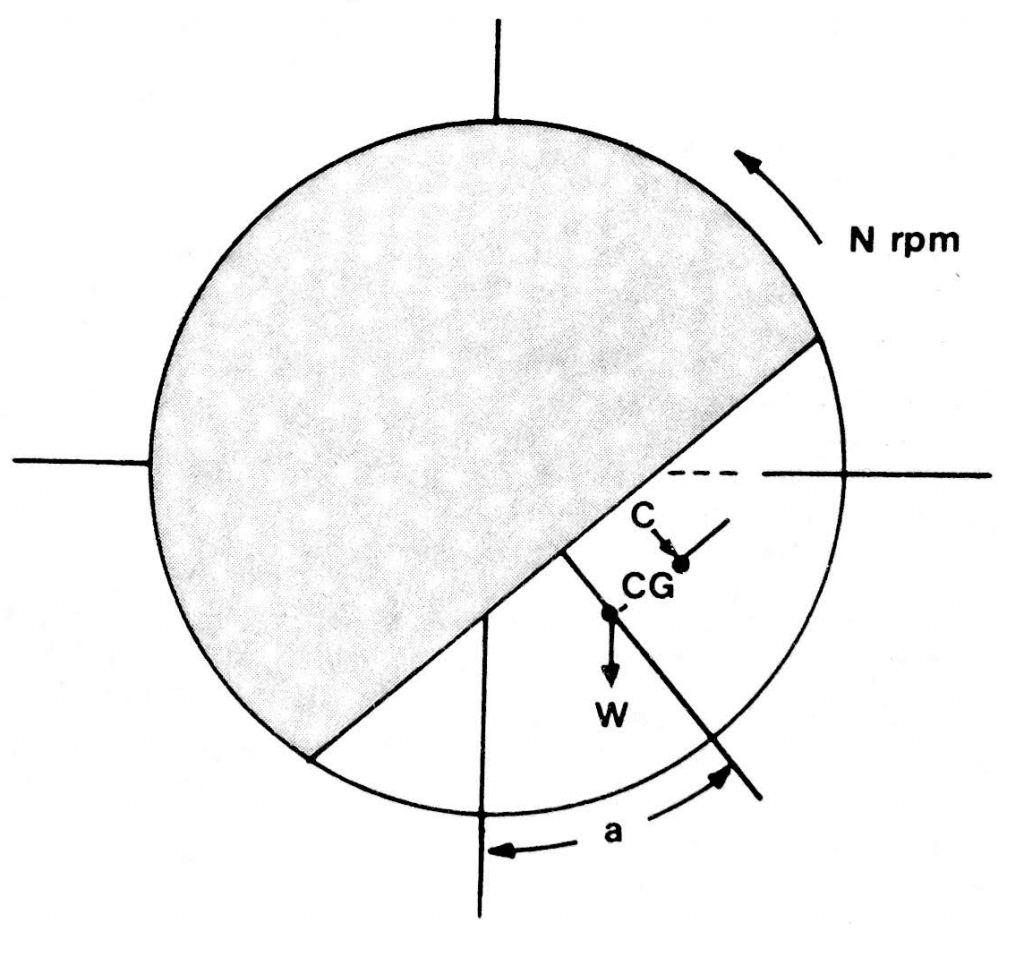The value of the angle “a” varies with the type of discharge, percent of critical speed, and grinding condition.   In order to use the preceding equation, it is necessary to have considerable data on existing installations. Therefore, this approach has been simplified as follows:

Five basics conditions determine the horsepower drawn by a mill:

1. Diameter
2. Length
4. Speed
5. Mill type

These conditions have been built into factors which are given in the figure above. The approximate horsepower HP of a mill can be calculated from the following equation:

HP = (W) (C) (Sin a) (2π) (N)/ 33000

where:

W = weight of charge
C = distance of centre of gravity or charge from centre of mill in feet
a = dynamic angle of repose of the charge
N = mill speed in RPM

HP = A x B x C x L

Where

A = factor for diameter inside shell lining
B = factor which includes effect of % loading and mill type
C = factor for speed of mill
L = length in feet of grinding chamber measured between head liners at shell- to-head junction

Many grinding mill manufacturers specify diameter inside the liners whereas others are specified per inside shell diameter. (Subtract 6” to obtain diameter inside liners.)  Likewise, a similar confusion surrounds the length of a mill. Therefore, when comparing the size of a mill between competitive manufacturers, one should be aware that mill manufacturers do not observe a size convention.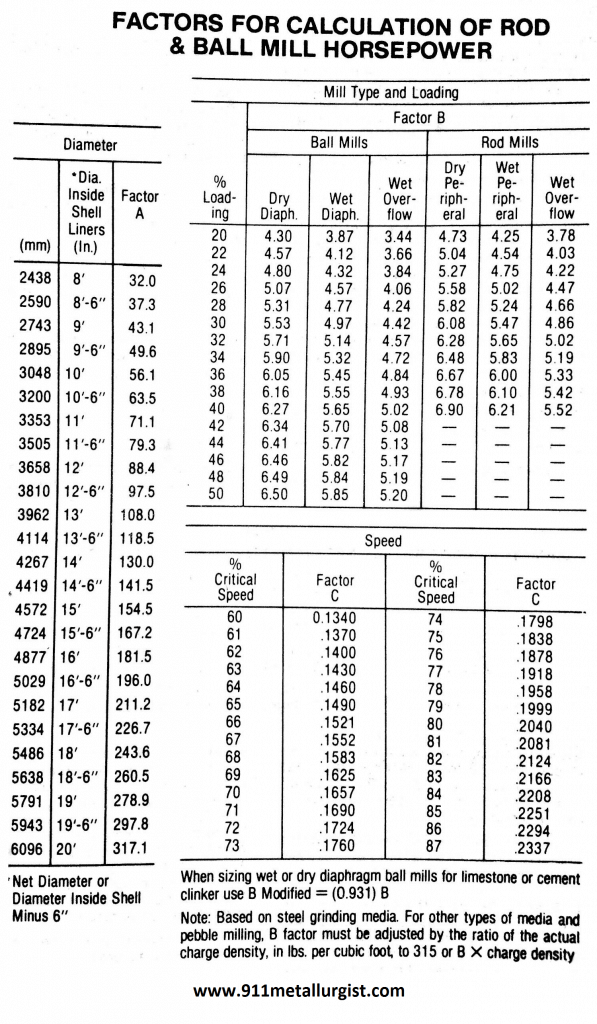Ball Mill Power/Design Calculation Example #2

In Example No.1 it was determined that a 1400 HP wet grinding ball mill was required to grind 100 TPH of material with a Bond Work Index of 15 (guess what mineral type it is) from 80% passing ¼ inch to 80% passing 100 mesh in closed circuit.  What is the size of an overflow discharge ball mill for this application?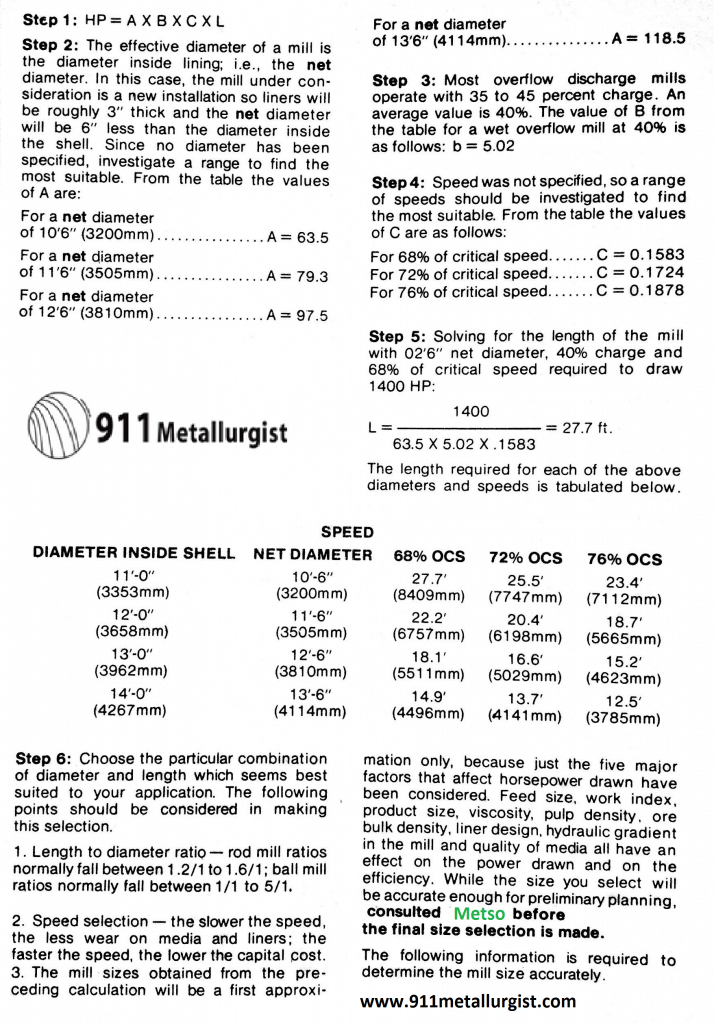Contact http://www.metso.com/industries/mining/ if you need a Large Ball Mill.

Small Ball Mill Capacity & Sizing Table